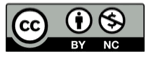# 双曲线

2018-06-10## 焦点定义

$$\{P | |PF_1| - |PF_2|= C\}$$## 解析定义

$${x^2 \over a^2}-{y^2 \over b^2}=1$$

$a$与$b$的比值决定了：

• 曲线开口的大小
• 渐近线的方程
• 焦点的位置## 精彩评论